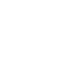# Top Rated Tutorials#B99AFF

In this tutorial, we look at two methods for creating a surface composed of planar quads.#2c3e50

This tutorial shows how to Unload and Load the PLA Filament on the back of the Makerbot.#3498db

In this tutorial we look at control flow for our scripts.#B99AFF

In part 2 of this tutorial sequence we look at simulating the tool along the toolpath to verify that its variable positional relationship#B99AFF

This tutorial is from my Intro to Computation and Fabrication class at CUNY.#3498db

In this quick tutorial we divide a surface up into points using python.#B99AFF

This tutorial introduces the class to the image map component.#42D692

In this tutorial, Brian shows how geometry can be made to travel along curves using motion paths.#3498db

In this tutorial we take a look at vectors and how to create simple object transformations using them.#CCA6AC

Yikes! This is the first tutorial I ever did with Grasshopper.#9FE1E7

This tutorial is by request. We add some options to export our models each trip through the loop.#7BD148

In this quick tutorial we apply what we learned about lists and loops from part 3 to create a list of points.

## Want to Contribute?

Want to be an author? Drop us a line here we'd love to have you.

Already have a video you'd like to post? Send us a link and we'll get you going.

:)